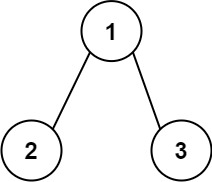304 North Cardinal St.
Dorchester Center, MA 02124

# Sum Root to Leaf Numbers LeetCode Solution

## Problem – Sum Root to Leaf Numbers LeetCode Solution

You are given the `root` of a binary tree containing digits from `0` to `9` only.

Each root-to-leaf path in the tree represents a number.

• For example, the root-to-leaf path `1 -> 2 -> 3` represents the number `123`.

Return the total sum of all root-to-leaf numbers. Test cases are generated so that the answer will fit in a 32-bit integer.

leaf node is a node with no children.

Example 1:``````Input: root = [1,2,3]
Output: 25
Explanation:
The root-to-leaf path 1->2 represents the number 12.
The root-to-leaf path 1->3 represents the number 13.
Therefore, sum = 12 + 13 = 25.
``````

Example 2:``````Input: root = [4,9,0,5,1]
Output: 1026
Explanation:
The root-to-leaf path 4->9->5 represents the number 495.
The root-to-leaf path 4->9->1 represents the number 491.
The root-to-leaf path 4->0 represents the number 40.
Therefore, sum = 495 + 491 + 40 = 1026.
``````

Constraints:

• The number of nodes in the tree is in the range `[1, 1000]`.
• `0 <= Node.val <= 9`
• The depth of the tree will not exceed `10`.

## Sum Root to Leaf Numbers LeetCode Solution in Java

``````public int sumNumbers(TreeNode root) {
return sum(root, 0);
}

public int sum(TreeNode n, int s){
if (n == null) return 0;
if (n.right == null && n.left == null) return s*10 + n.val;
return sum(n.left, s*10 + n.val) + sum(n.right, s*10 + n.val);
}
``````

## Sum Root to Leaf Numbers LeetCode Solution in C++

``````class Solution {
public:
int sumNumbers(TreeNode* root, int num=0) {
return root->left == root->right ? num * 10 + root->val :
((root->left ? sumNumbers(root->left, num * 10 + root->val) : 0) +
(root->right ? sumNumbers(root->right, num * 10 + root->val) : 0));
}
};
``````

## Sum Root to Leaf Numbers LeetCode Solution in Python

``````def sumNumbers(self, root):
"""
:type root: TreeNode
:rtype: int
"""
def helper(sum, root):
if not root:
return 0
sum = sum * 10 + root.val
if not root.left and not root.right:
return sum
return helper(sum, root.left) + helper(sum, root.right)

return helper(0, root)
``````
##### Sum Root to Leaf Numbers LeetCode Solution Review:

In our experience, we suggest you solve this Sum Root to Leaf Numbers LeetCode Solution and gain some new skills from Professionals completely free and we assure you will be worth it.

If you are stuck anywhere between any coding problem, just visit Queslers to get the Sum Root to Leaf Numbers LeetCode Solution

Find on Leetcode

##### Conclusion:

I hope this Sum Root to Leaf Numbers LeetCode Solution would be useful for you to learn something new from this problem. If it helped you then don’t forget to bookmark our site for more Coding Solutions.

This Problem is intended for audiences of all experiences who are interested in learning about Data Science in a business context; there are no prerequisites.

Keep Learning!

More Coding Solutions >>

LeetCode Solutions

Hacker Rank Solutions

CodeChef Solutions